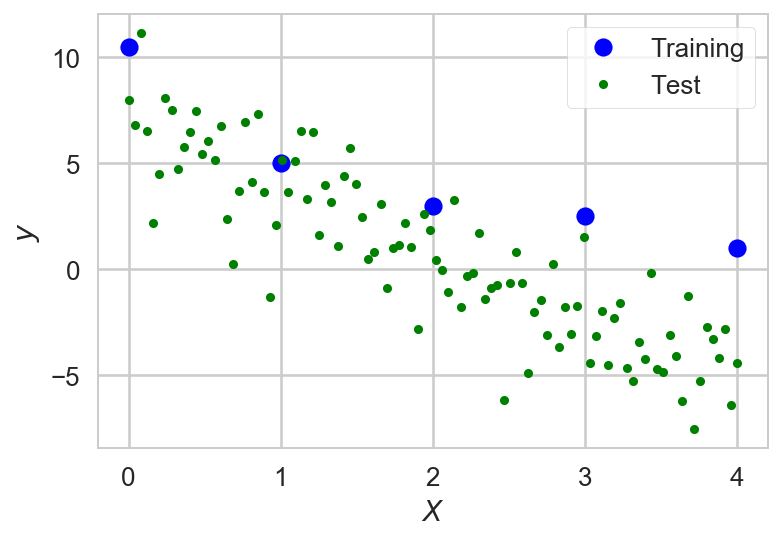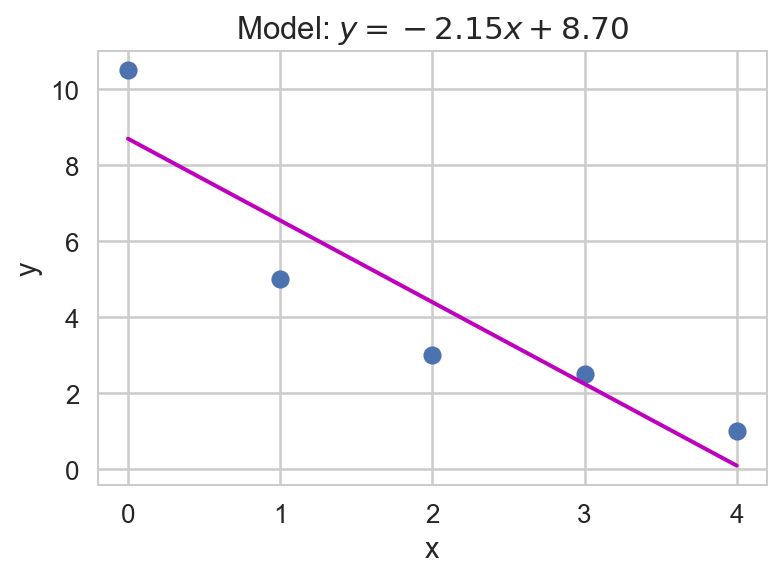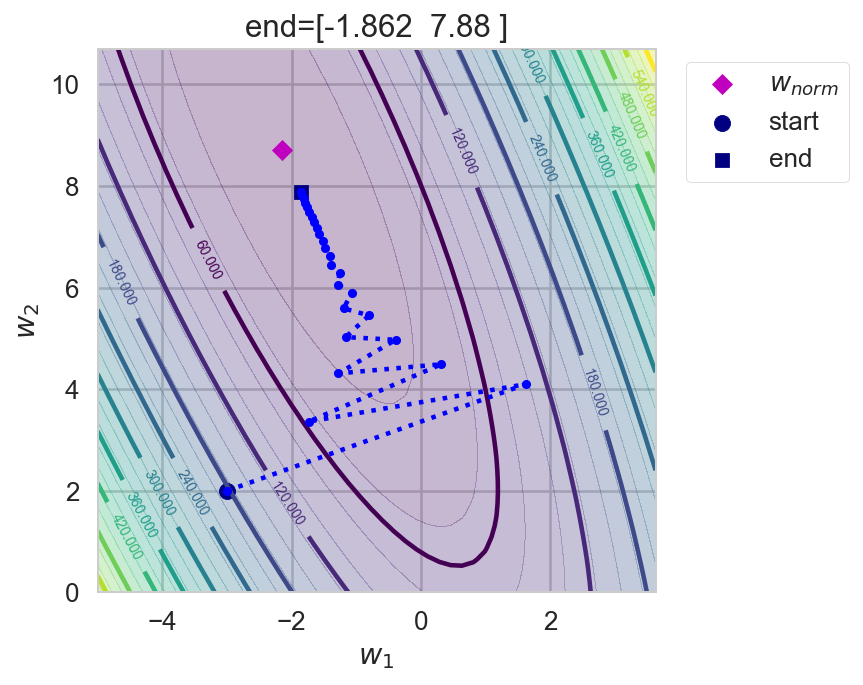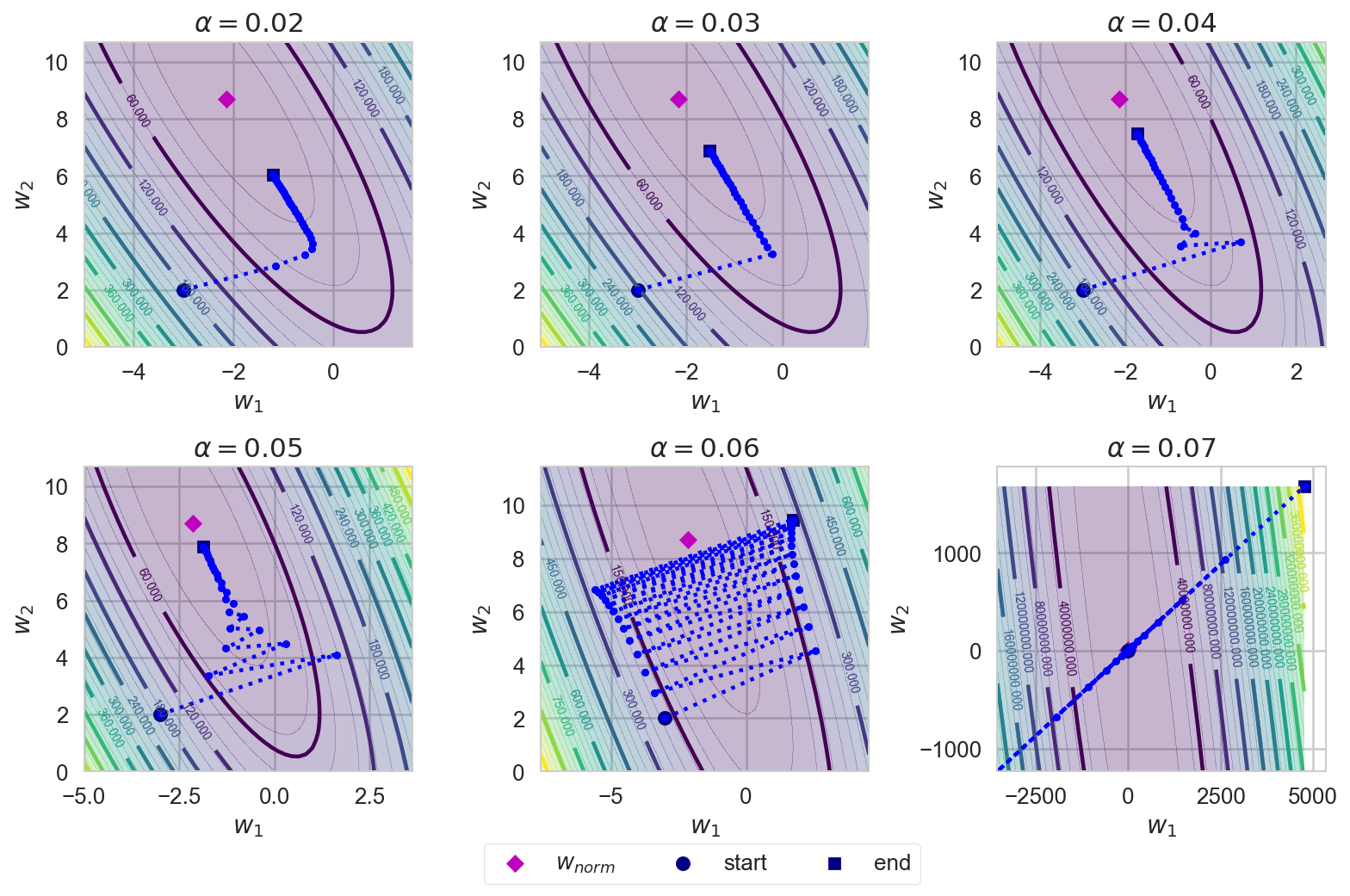# Regression$\renewcommand{\vec}{\boldsymbol{#1}}$¶

• We are already familiar with regression problems.
• We have a dataset $\Psi=\left\{\left<\vec{x}^{(1)},\vec{y}^{(1)}\right>,\ldots,\left<\vec{x}^{(i)},\vec{y}^{(i)}\right>,\ldots\left<\vec{x}^{(m)},\vec{y}^{(m)}\right>\right\}$ of noisy samples drawn from and unknown function $\vec{F}:\mathcal{D}\rightarrow\mathcal{I}$.
• In the regression case $\mathcal{D}\subseteq\mathbb{R}^n$ and $\mathcal{I}\subseteq\mathbb{R}^k$.

We can assume that $k=1$ and noise is Gaussian and that $\Psi$ is finite.

### Why we use the Gaussian noise assumption¶

We will assume that the target variable is described by

$$y = \vec{F}(\vec{x},\vec{w}) + \varepsilon$$

where:

• $\vec{F}(\vec{x},\vec{w})$ is a (possibly undefined and/or unknown) function of $\vec{x}$ and $\vec{w}$ and
• $\varepsilon\sim\mathcal{N}(0,\sigma^2_\varepsilon)$ is a Gaussian-distributed noise component.

#### Gaussian Noise?¶

The derivations provided below all assume Gaussian noise on the target data. Is this a good assumption? In many cases yes. The argument hinges on the use of the Central_Limit_Theorem that basically says the the sum of many independent random variables behaves behaves like a Gaussian distributed random variable.

The noise term in this model, $\epsilon$, can be thought of as the sum of features not included in the model function, $\vec{F}(\vec{x},\vec{w})$. Assuming these features are themselves independent random variables then the Central Limit Theorem suggests a Gaussian model is appropriate, assuming there are many independent unaccounted for features.

It is possible that there is only a small number of unaccounted for features or that there is genuine non-Gaussian noise in our observation measurements, e.g. sensor shot noise that often has a Poisson distribution. In such cases, the assumption is no longer valid.

# An Example¶

Training dataset

In :
N = 5                                              # Size of the data set
X = np.array([[0.0], [1.0], [2.0], [3.0], [4.0]])  # Inputs, shape: N x 1
y = np.array([10.5, 5.0, 3.0, 2.5, 1.0])           # Outputs, shape: N

In :
X.T

Out:
array([[ 0.,  1.,  2.,  3.,  4.]])
In :
y

Out:
array([ 10.5,   5. ,   3. ,   2.5,   1. ])

Test dataset

In :
N_test = 100
X_test = np.linspace(0.0, 4.0, N_test).reshape((N_test, 1))
y_test = np.linspace(7.0, -5.0, N_test)+ 2*np.random.randn(N_test)


### Ploting the training and test datasets¶

In :
plt.plot(X[:, 0], y, 'bo', label='Training')
plt.plot(X_test[:, 0], y_test, "g.", label='Test')
plt.xlabel('$X$'); plt.ylabel('$y$'); plt.legend(frameon=True);# Linear Regression Model¶

• We can express our constraints down with a linear model:
$$y^{(i)} + \varepsilon^{(i)} = \vec{x}^{(i)}\cdot \vec{w} + b, \forall i \in \{1,\ldots,m\}\,,$$

where $\vec{w}$ and $b$ constitute a weight vector and $\varepsilon^{(n)} \sim \mathcal{N}(0, \sigma^2_\varepsilon)$.

• Weights are parameters of the linear model that can be tuned to fit the training data better.

A shorter version of this expression is the following equation:

$$\vec{y} + \vec{\epsilon} = \vec{X} \cdot \vec{w},\ \vec{X} \in \mathbb{R}^{m \times n},\ \vec{y} \in \mathbb{R}^m,\ \vec{\epsilon} \in \mathbb{R}^m,\ \vec{w} \in \mathbb{R}^n,$$

where the $i$-th row of $\vec{X}$ represents $\vec{x}^{(i)}$ and the $j$-th entry of the vector $\vec{y}$ represents $\vec{y}^{(j)}$.

• It might not be possible to find a weight vector that satisfies the constraints perfectly.
• Instead, we can minimize the sum of squared errors (SSE):
$$\hat{\vec{w}} = \text{arg}\,\text{min}_\vec{w} \dfrac{1}{2}\left\|\vec{X}\vec{w} - \vec{y}\right\|^2_2,$$

where $\left\|\vec{A}\right\|_2$ is called Frobenius norm and is the generalization of the Euclidean norm for matrices.

## Learning Weights¶

There are two ways to adjust the weights of a linear model (which includes linear models with nonlinear features):

#### Normal equations (analytical solution)¶

• Requires inversion of $m \times n$ matrix.
• It does not scale well with the number of features.

• Requires the calculation of $m$ gradients in each step.
• It does not scale well with the number of examples.

In addition, we can add a constraint to the objective function to penalize large weights: Tikhonov regularization (forward pointer).

## Normal Equations¶

Solving $\vec{X} \cdot \vec{w} = \vec{y}$ means solving $$\left\{ \begin{array}{rcrcl} x^{(1)}_1 w_1 & + x^{(1)}_2 w_2 & + \cdots & + x^{(1)}_n w_n & = y^{(1)} \\ x^{(2)}_1 w_1 & + x^{(2)}_2 w_2 & + \cdots & + x^{(2)}_n w_n & = y^{(2)} \\ x^{(3)}_1 w_1 & + x^{(3)}_2 w_2 & + \cdots & + x^{(3)}_n w_n & = y^{(3)} \\ \vdots & \vdots & \ddots & \vdots & = \vdots \\ x^{(m)}_1 w_1 & + x^{(m)}_2 w_2 & + \cdots & + x^{(m)}_n w_n & = y^{(m)} \end{array} \right.\,.$$

• Frequently, solving equation $\vec{X} \cdot \vec{w} = \vec{y}$ directly for $\vec{w}$ by inversion is not viable.
• As $\vec{X}$ is not necessarily a square matrix, therefore $\vec{w} = \vec{X}^{-1} \vec{y}$ is usually not possible.
• This implies that it is usually impossible to find an exact solution.
• These systems are overdetermined.

### The linear least squares solution¶

• The linear least squares is the problem of approximately solving an overdetermined system of linear equations.
• The best approximation is defined as that which minimizes the sum of squared differences between the data values and their corresponding modeled values.

That is, the solution of

$$\vec{X}^\intercal\vec{X} \hat{\boldsymbol{w}} = \vec{X}^\intercal\vec{y}$$

for $\hat{\boldsymbol{w}}$, i.e.,

$$\hat{\vec{w}} = (\vec{X}^\intercal\vec{X})^{-1}\vec{X}^\intercal\vec{y}.$$
In :
def normal_equations(X, y):
# w = np.linalg.inv(X.T.dot(X)).dot(X.T).dot(y)
# ... or we can use the Moore-Penrose pseudoinverse (is usually more likely to
# be numerically stable)
w = np.linalg.pinv(X).dot(y)
# ... or we use the solver
# w = np.linalg.lstsq(X, y)
return w


### The bias trick¶

In :
X_bias = np.hstack((X, np.ones((N, 1))))
X_test_bias = np.hstack((X_test, np.ones((N_test, 1))))

In :
X.T

Out:
array([[ 0.,  1.,  2.,  3.,  4.]])
In :
X_bias.T

Out:
array([[ 0.,  1.,  2.,  3.,  4.],
[ 1.,  1.,  1.,  1.,  1.]])
In :
w_norm = normal_equations(X_bias, y)

In :
plt.plot(X_bias[:, 0], y, "o")
plt.plot(X_bias[:, 0], X_bias.dot(w_norm), "m-", linewidth=2)
plt.xlabel("x"); plt.ylabel("y"); plt.title("Model: $y = %.2f x + %.2f$" % tuple(w_norm));We can now solve linear regression problems at will!

## Homework 1¶

Test the other forms of normal equations.

• Check the numerical stability of each approach and
• how long they take to compute.

## Computational cost and complexity¶

Think of this problem:

• inputs: 100 color images of size 256x256 pixels
• output: radius of the ball which is at the center of the image
• Is the direct solution via normal equations viable?

## Estimating problem tractability¶

Assuming that each pixel is represented by three bytes defining the RGB values:

In :
n_pixels = 256 * 256
memory_size_xtx = n_pixels * n_pixels * 3 * 8
print("Required memory: %d GB" % (memory_size_xtx / 2**30))

Required memory: 96 GB


Inversion is an procedure that requires $O(n^{2.373})$ operations (Source).

In cases where the training data set is very large or data is received in a stream, a direct solution using the normal equations may not be possible. An alternative approach is the stochastic gradient descent algorithm.

Iteratively update the weights using the gradient as reference.$\Delta \vec{w}_t$ is minus the derivative of the error function $E(\vec{X}, \vec{y};\vec{w}_t)$ with respect to the weight $w_i$:

$$\Delta w_i = - \alpha\frac{\partial E(\vec{X}, \vec{y};\vec{w})}{\partial w_i}\,,$$

where:

• $\alpha$ is a hyperparameter called learning rate, it has to be set manually and must usually be within $\left(0, 1\right)$, typical values are $10^{-1}$, $10^{-2}$, $10^{-3}$, ...

Update $\vec{w}$ incrementally in every iteration $t$ as:

$$\vec{w}(t+1) = \vec{w}(t) + \Delta \vec{w}(t)\,,$$

### Forward pointer¶

• Gradient descent actually is a more general rule.
• It can be applied to any minimization problem if the gradient can be computed.
• For example, artificial neural networks, support vector machines, $k$-means, etc.

## Gradient of the Linear Model¶

Using as error function the sum of squared errors (SSE),

$$E(\boldsymbol{X}, \boldsymbol{y};\boldsymbol{w}) = \frac{1}{2}\left\|\boldsymbol{X} \cdot \boldsymbol{w} - \boldsymbol{y}\right\|^2_2\,.$$

$$\nabla \boldsymbol{w} = \nabla_{\boldsymbol{w}} E(\boldsymbol{X}, \boldsymbol{y};\boldsymbol{w}) = \boldsymbol{X}^T \cdot (\boldsymbol{X} \cdot \boldsymbol{w} - \boldsymbol{y})\,.$$

In :
def error(X, y, w):
return 0.5*np.linalg.norm(X.dot(w) - y)**2

In :
def linear_regression_gradient(X, y, w):
return X.T.dot(X.dot(w)-y)


## The learning loop¶

Initialize weights to small random values

In :
w = np.random.random(2)/10000

In :
alpha = 0.05

In :
for i in range(50):
# print('Iteration', i+1, 'weights', w, 'error', error(X_bias, y, w))
w = w - alpha*linear_regression_gradient(X_bias, y, w)
print('w_norm:', w_norm, 'w grad. desc.', w)

w_norm: [-2.15  8.7 ] w grad. desc. [-2.089  8.526]


Implementing the gradient descent loop as a function.

In :
def gradient_descent(X, y, w_0, alpha, max_iters):
'Returns the values of the weights as learning took place.'
w = np.array(w_0, dtype=np.float64)
w_hist = np.zeros(shape=(max_iters+1, w.shape))
w_hist = w
for i in range(0,max_iters):
w += delta_weights
w_hist[i+1] = w
return w_hist

In :
w_0 = [-3,2] # we fix the initial weights to make it more illustrative
alpha = 0.05
max_iters = 25

In :
w_hist = gradient_descent(X_bias, y, w_0, alpha, max_iters)

In :
plot_hist_contour(X_bias, y, w_hist, w_norm, title='end='+str(w_hist[-1]), show_legend=True)What is the impact of the learning rate ($\alpha$)?

In :
def alphas_study(alphas):
fig = plt.figure(figsize=(11,7))
for i,alpha in enumerate(alphas):
w_hist = gradient_descent(X_bias, y , w_0, alpha, max_iters)
plot_hist_contour(X_bias, y, w_hist, w_norm, ax=ax, title='$\\alpha='+str(alpha)+'$')
plt.legend(scatterpoints=1, ncol=3, bbox_to_anchor=(-0.2,-0.2), frameon=True);
plt.tight_layout()


## Impact of learning rate¶

In :
alphas = np.linspace(0.02,0.07,6)
alphas

Out:
array([ 0.02,  0.03,  0.04,  0.05,  0.06,  0.07])
In :
alphas_study(alphas)### Improving GD by adding a momentum term¶

Idea adding some an inertial momentum to the process can:

• accelerate convergence when moving in a plateau, or
• damp oscilations.

Update $\vec{w}$ incrementally in every iteration $t$ as:

$$\vec{w}(t+1) = \vec{w}(t) + \alpha\Delta \vec{w}(t) + \beta \Delta \vec{w}(t-1),$$

where $\beta\in\mathbb{R}^+$ is known as the momentum rate.

In :
def gradient_descent_with_momentum(X, y, w_0, alpha, beta, max_iters):
w = np.array(w_0, dtype=np.float64)
w_hist = np.zeros(shape=(max_iters+1, w.shape))
w_hist = w
omega = np.zeros_like(w)
for i in range(max_iters):
delta_weights = -alpha*linear_regression_gradient(X, y, w) + beta*omega
omega = delta_weights
w += delta_weights
w_hist[i+1] = w
return w_hist

In :
alpha = 0.05
beta = 0.5
max_iters = 25

In :
w_hist = gradient_descent(X_bias, y, (-3,2), alpha, max_iters)
w_hist_mom = gradient_descent_with_momentum(X_bias,y, (-3,2), alpha, beta, max_iters)

In :
comparison_plot()